Time Value of Money | IFT World
IFT Notes for Level I CFA® Program
IFT Notes for Level I CFA® Program

# Part 2

## 3. Future Value of a Single Cash Flow

Let’s understand this concept with a simple example.

Say present value (PV) = $100 and interest rate (r) = 10%. What is the future value (FV) after one year? What is the future value (FV) after two years? The future value of a single cash flow can be computed using the following formula: FVN = PV(1 + r)N where: FVN = future value of the investment N = number of periods PV = present value of the investment r = rate of interest Therefore, FV1 = 100(1 + 0.1)1 =$110
FV2 = 100(1 + 0.1)2 = $121 Notice that with compound interest, after two years we have$121. Whereas, with simple interest, after two years we would have $120. The difference between the two values ($1) represents the interest-on-interest component. In Year 2, we not only receive interest on the $100 principal, but we also receive interest on the$10 interest earned in Year 1 that has been reinvested.

Example

Cyndia Rojers deposits $5 million in her savings account. The account holders are entitled to a 5% interest. If Cyndia withdraws cash after 2.5 years, how much cash would she most likely be able to withdraw? Solution: FVN = PV(1 + r)N FV2.5 = 5(1 + 0.05)2.5 =$5.649 million

FV Calculation using a Financial Calculator

You will often use the following keys on your TI BA II Plus calculator:

N = number of periods

I/Y = rate per period

PV = present value

FV = future value

PMT = payment

CPT = compute

One important point to note is the signs used for PV and FV. If the value for PV is negative “-”, then the value for FV is positive “+”. An inflow is often represented as a positive number, while outflows are denoted by negative numbers.

Before you begin, set the number of decimal points on your calculator to 9 to increase accuracy.

 Keystrokes Explanation Display [2nd] [FORMAT] [ ENTER ] Get into format mode DEC = 9 [2nd] [QUIT] Return to standard calc mode 0

Question: You invest $100 today at 10% compounded annually. How much will you have in 5 years? The key strokes to compute the future value of a single cash flow are illustrated below.  Keystrokes Explanation Display [2nd] [QUIT] Return to standard calc mode 0 [2nd] [CLR TVM] Clears TVM Worksheet 0 5 [N] Five years/periods N = 5 10 [I/Y] Set interest rate I/Y = 10 100 [PV] Set present value PV = 100 0 [PMT] Set payment PMT = 0 [CPT] [FV] Compute future value FV = -161.05 ## 4. Non-Annual Compounding (Future Value) When our compounding frequency is not annual, we use the following formula to compute future value: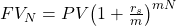where: rs = the stated annual interest rate in decimal format m = the number of compounding periods per year N = the number of years Let’s understand this concept using an example. You invest$80,000 in a 3-year certificate of deposit. This CD offers a stated annual interest rate of 10% compounded quarterly. How much will you have at the end of three years?

Solution:

There are two methods to solve this question.

Formula Method

PV is $80,000. The stated annual rate is 10%. The number of compounding periods per year is 4. The total number of periods is 4 x 3 = 12. Therefore future value after 12 quarters (3 years) is FV12 =$80,000(1 + 0.025)12 = $107,591 Calculator Method You can also solve this problem using a financial calculator; the key strokes are given below: N = 12, I/Y = 2.5%, PV =$80,000, PMT = 0, CPT FV = -$107,591 PMT is 0 because there are no intermediate payments in this example. Example Donald invested$3 million in an American bank that promises to pay 4% compounded daily. Which of the following is closest to the amount Donald receives at the end of the first year? Assume 365 days in a year.

1. A. $3.003 million 2. B.$3.122 million
3. C. $3.562 million Solution The correct answer is B. Formula Method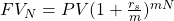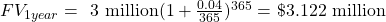Calculator Method N = 365, I/Y = 4/365%, PV =$3 million, PMT = 0; CPT FV = -$3.122 million ## 5. Continuous Compounding We saw examples with annual compounding. Then we discussed quarterly compounding and in the above example we looked at daily compounding. If we keep increasing the number of compounding periods until we have infinite number of compounding periods per year, then we can say that we have continuous compounding. The formula for computing future values with continuous compounding is: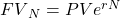where: r = continuously compounded rate N = the number of years Let’s look at an example. An investment worth$50,000 earns interest that is compounded continuously. The stated annual interest is 3.6%. What is the future value of the investment after 3 years?

Solution:

PV = $50,000; r = 0.036; N = 3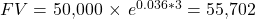Concept Building Exercise Assume that the stated annual interest rate is 12%. What is the future value of$100 at different compounding frequencies? What is the return?

 Frequency Future value of $100 Return Annual 112.00 12.00% Semiannual 112.36 12.36% Quarterly 112.55 12.55% Monthly 112.68 12.68% Daily 112.75 12.75% Continuous 112.75 12.75% Instructor’s Note: 1. 1. For the same stated annual rate, the returns keep getting better as we compound more often. 2. 2. If you have two banks that offer the following rates 3. A: 12.5% compounded annually 4. B: 12% compounded daily Which offer is better? Even though A’s 12.5% looks better, B’s 12% compounded daily will effectively give you a return of 12.75%. Therefore the offer from bank B is better. ### 5.1. Stated and Effective Rates Now we come to the related concept of stated versus effective rates. In the above concept-building exercise, the stated rate was 12% across the board, but the effective rate that an investor actually earns depends on the compounding frequency. The effective rates were different for different compounding frequencies. If we are given a compounding frequency, we can compute effective rates using the following formulae: Effective annual rate for discrete compounding: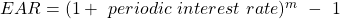where: m = number of compounding periods in one year • For daily compounding, m = 365 • For monthly compounding, m = 12 • For quarterly compounding, m = 4 • For semiannual compounding, m = 2 For example, for a stated annual rate of 12% and quarterly compounding, the EAR will be equal to: EAR = (1 + 0.12/4)4 – 1 = 1.1255 – 1 = 0.1255 = 12.55% Instructor’s Note: A lot of people get confused about the -1 at the end of the formula. The idea is actually fairly straight forward. Basically we have 1.034 which is telling us how much$1 will become at the end of 4 periods. $1 is going to become$1.1255. But this is not a rate. To figure out the rate we have to subtract the original $1 that we invested. So we are left with 0.1255 which is our effective rate. Effective Annual Rate for continuous compounding: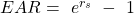where rs = stated annual interest rate For example, for a stated annual rate of 12% and continuous compounding, the EAR will be equal to: EAR = e0.12 – 1 = 1.1275 – 1 = 0.1275 = 12.75% ## 6. Series of Cash Flows The different types of cash flows based on the time periods over which they occur include: • Annuity: A finite set of equal sequential cash flows. The two types are • Ordinary annuity: The first cash flow occurs one period from now (indexed at t = 1). • Annuity due: The first cash flow occurs immediately (indexed at t = 0). • Perpetuity: A set of equal never-ending sequential cash flows with the first cash flow occurring one period from today. (The period is finite in case of an annuity whereas in perpetuity it is infinite.) ### 6.1. Equal Cash Flows – Ordinary Annuity Let’s say that we have an ordinary annuity with A =$1,000, r = 5% and N = 5, i.e. we are going to receive a payment of $1,000 at the end of each year for the next 5 years and our discount rate is 5%. We can compute the FV of this annuity using the following three methods: Brute-Force Method We can take each individual cash flow and see how much each of these will be worth at the end of five years. Then we can add all the values to compute the total FV of the annuity. For instance, the first$1,000 deposit made at t = 1 will compound over four periods; the second deposit of $1,000 will compound over three periods and so on. We then add the future values of all payments to arrive at the future value of the annuity which is$5,525.63.

Formula Method

The future value of an annuity can also be computed using the following formula: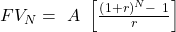where:

A = annuity amount

N = number of years

The term in square brackets is known as the ‘future value annuity factor’ (FVAF). This factor gives the future value of an ordinary annuity of $1 per period. Hence the formula given above can also be written as: FV = A x FVAF. Therefore, using the formula: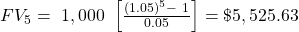Calculator Method Given below are the keystrokes for computing the future value of an ordinary annuity.  Keystrokes Explanation Display [2nd] [QUIT] Return to standard calc mode 0 [2nd] [CLR TVM] Clears TVM Worksheet 0 5 [N] Five years/periods N = 5 5 [I/Y] Set interest rate I/Y = 5 0 [PV] 0 because there is no initial investment PV = 0 1000 [PMT] Set annuity payment PMT = 1000 [CPT] [FV] Compute future value FV = -5525.63 On the exam you should use the calculator method, because this is the fastest method and does not require you to memorize the annuity formula. Example Haley deposits$24,000 in her bank account at the end of every year. The account earns 12% per annum. If she continues this practice, how much money will she have at the end of 15 years?

Solution:

N = 15, I/Y = 12%, PV = 0, PMT = $24,000; CPT → FV = -$894,713.15

### 6.2. Unequal Cash Flows

If cash flow streams are unequal, the future value annuity factor cannot be used. In this case, we find the future value of a series of unequal cash flows by compounding the cash flows one at a time.

This concept is illustrated in the figure below. We need to find the future value of five cash flows: $1,000 at the end of Year 1;$2,000 at the end of Year 2; $3,000 at the end of Year 3;$4,000 at the end of Year 4; and $5,000 at the end of Year 5. The future value is$5,000 + $4,000 x 1.05 +$3,000 x 1.052 +$2,000 x 1.053 +$1,000 x 1.054 = \$16,038.